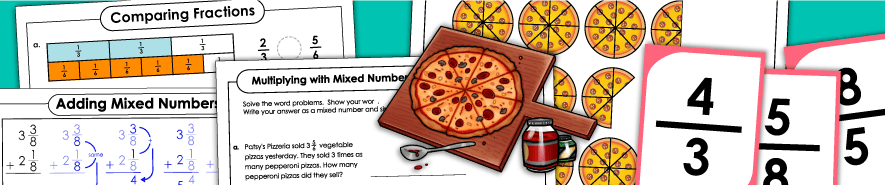# Fraction Worksheets

Several different types of fraction worksheets are available on the pages below. Includes basic fraction worksheets, equivalent fractions, comparing fractions, ordering fractions, and more.Fractions (Basic)

Printable fraction games and printable worksheets; Manipulative fraction strips, printable fraction pizzas, a memory-matching game, and more.

Equivalent Fractions & Simplifying

This page has worksheets and activities for teaching students about equivalent fractions and reducing fractions into simplest terms.

Comparing & Ordering Fractions

Compare and order pairs of fractions with these task cards, learning center activities, and worksheets.

Fractions on Number Lines

These worksheets all feature fractions on number lines.

Fractional Parts of a Set

Download and print activities on calculating fractional parts of sets. (example: What is 3/4 of 24?)

Mixed Numbers & Improper Fractions

Mixed number printables; Includes basic mixed numbers, as well as adding and subtracting mixed numbers.

Reciprocal Fractions

Print worksheets for learning about reciprocal fractions.

## Operations With Fractions

Practice addition and subtraction of fractions (with same and different denominators) and mixed numbers.

Subtracting Fractions

Practice subtracting fractions and mixed numbers with these printables. Includes like and unlike denominators.

Multiplying Fractions

Multiply fractions and mixed numbers. Includes word problems, as well as worksheets with models and illustrations.

Dividing Fractions

With these worksheets, your students can practice dividing fractions and mixed numbers.

## More Fractions

Fraction Strips & Circles

This page has printable fraction strips, fraction circles, fraction pizzas, and other manipulative fraction tools.

Fractions: Halves & Quarters Only (Very Basic)

These very basic fraction worksheets cover only halves and quarters (fourths).

Least Common Denominators - LCD

Find the least common denominators for the pairs of fractions given.

Decimals

Learn about basic decimals, decimal place value, comparing and ordering decimals, rounding decimals, and adding, subtracting, multiplying, and dividing decimals.

Percentages

Learn about percentages, such as converting decimals and fractions to percents and word problems involving percents.

Ratios

Practice comparing two quantities with ratios. Look at the pictures and determine the ratios of objects.